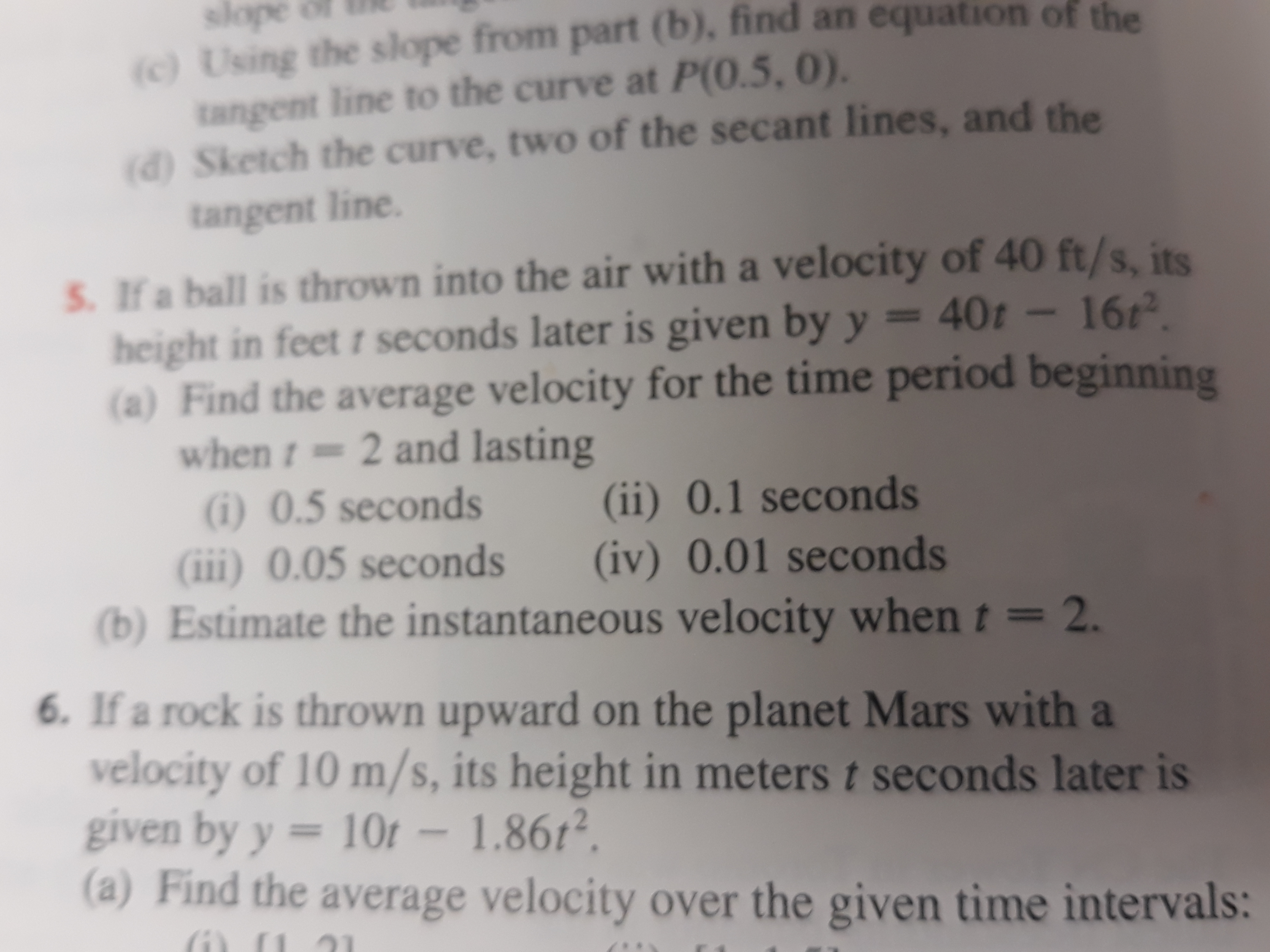# c) Using the slope from part (b), find an equation ofa) Sketch the curve, two of the secant lines, and theS. If a ball is thrown into the air with a velocity of 40 ft/s, itstangent line to the curve at P(0.5, 0).tangent line.height in feet t seconds later is given by y - 40t16(a) Find the average velocity for the time period beginningwhen 2 and lasting6) 0.5 seconds (ii) 0.1 seconds(ii) 0.05 seconds (iv) 0.01 seconds(b) Estimate the instantaneous velocity when t-26. If a rock is thrown upward on the planet Mars with avelocity of 10 m/s, its height in meters t seconds later isgiven by y -10r -1.8612(a) Find the average velocity over the given time intervals:

Question
30 viewshelp_outlineImage Transcriptionclosec) Using the slope from part (b), find an equation of a) Sketch the curve, two of the secant lines, and the S. If a ball is thrown into the air with a velocity of 40 ft/s, its tangent line to the curve at P(0.5, 0). tangent line. height in feet t seconds later is given by y - 40t 16 (a) Find the average velocity for the time period beginning when 2 and lasting 6) 0.5 seconds (ii) 0.1 seconds (ii) 0.05 seconds (iv) 0.01 seconds (b) Estimate the instantaneous velocity when t-2 6. If a rock is thrown upward on the planet Mars with a velocity of 10 m/s, its height in meters t seconds later is given by y -10r -1.8612 (a) Find the average velocity over the given time intervals: fullscreen
check_circle

Step 1

Given: y=40t-16t^2

Step 2

a.i) Average velocity between 2 and 2.5 sec=-32 ft/s

Step 3

a.ii) Average velocity between ...

### Want to see the full answer?

See Solution

#### Want to see this answer and more?

Solutions are written by subject experts who are available 24/7. Questions are typically answered within 1 hour.*

See Solution
*Response times may vary by subject and question.
Tagged in

### Calculus